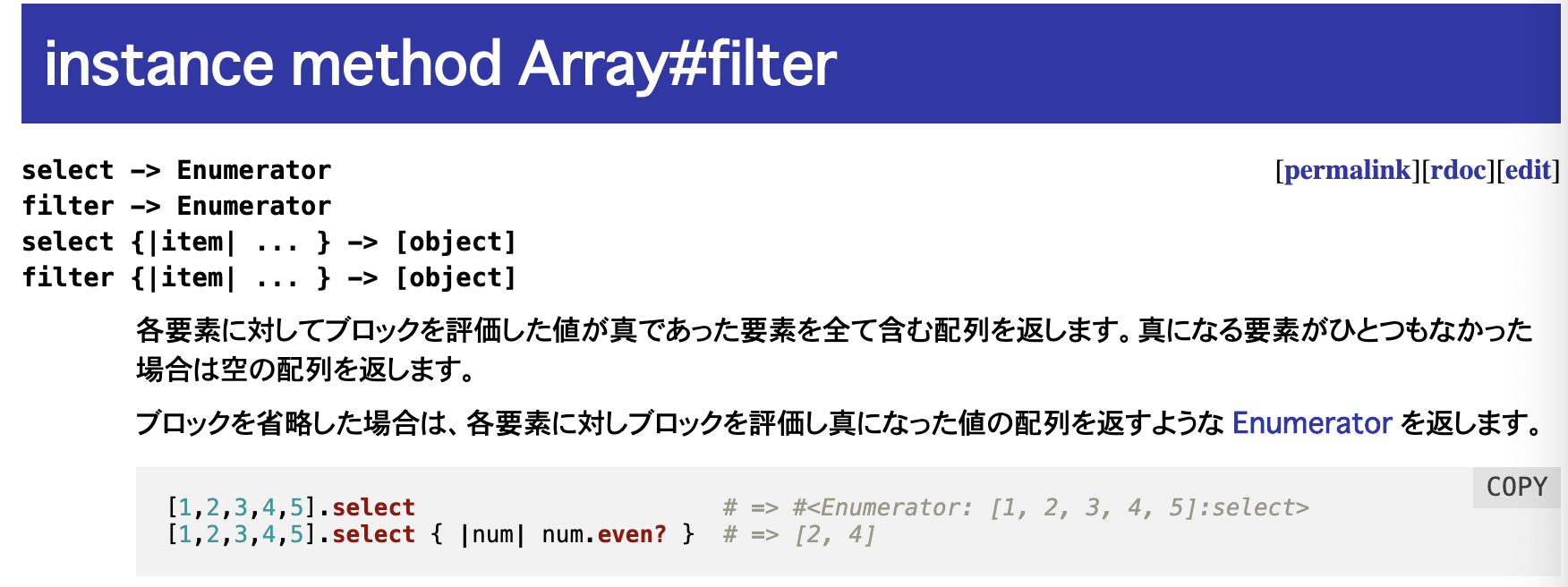# [Ruby] Extract only the elements that match the conditions and create a new array. filter and select

This is a personal memo.

There are `select` and` filter` as methods to extract only the elements that match the conditions and create a new array.

This is the same for both. Looking at the official page of ruby, select is used in the example of the filter method.Ruby Official Array # filter

## range object For range objects from 1 to 3, only the numerical values ​​corresponding to 2 or less are extracted and an array is created.

#### `select`

``````
range = 1..3   #[1,2,3]
range2 = range.select{|x| x<=2}

p range2
#output
[1, 2]
``````

#### `filter`

``````
range = 1..3   #[1,2,3]
range2 = range.filter{|x| x<=2}

p range2
#output
[1, 2]
``````

The result is the same for both filter and select.

## Array

#### `select`

``````
arr = [1,2,3]
arr2 = arr.select{|x| x<=2}

p arr2
#output
[1, 2]
``````

## object For objects, set two variables. The first variable is the key and the second variable is the value.
• If there is only one variable, a KV pair will be entered.

#### `python`

``````
obj = {:a=>1, :b=>2, :c=>3}
obj2 = obj.select{|x, y| y<=2}

p obj2
#output
{:a=>1, :b=>2}
``````

Unlike the map method, the processing result is returned as an object. (In the case of map, an array is returned)